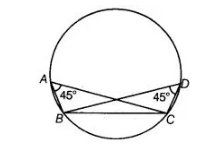# If A, B, C and D are four points such that`
Question:

If A, B, C and D are four points such that ∠BAC = 45° and ∠BDC = 45°, then A, B, C and D are concyclic.

Solution:

True

Since, ∠BAC = 45° and ∠BDC = 45°As we know, angles in the same segment of a circle are equal. Hence, A, B, C and D are concyclic.